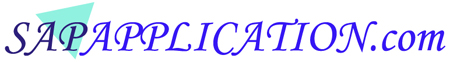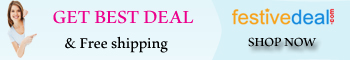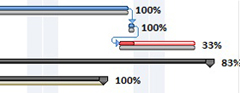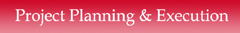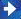Continue.. EVM - Project Estimation and Project Variance   9. Estimate At Completion (EAC) - EAC is the projection of the total cost at the end of       the project. It is the actual cost occurred till date plus an estimated cost to complete       the remaining work. The EAC is calculated as - EAC = Actual Cost (AC) + Estimate to Complete (ETC)           There are different methods to calculate EAC. A project can select the formula           that best fits as per their project type, complexity and size. The EAC can also           be calculated as -           EAC = BAC / CPI = BAC*AC / EV. EAC = AC + (BAC - EV) / CPI                    The EAC should be evaluated periodically on a monthly basis or when any           major changes happens in the project. 10. Estimate To Complete (ETC) - ETC is the estimated cost to complete the remaining       work of the project. ETC needs to be evaluated again if any significant changes       happens and the previous estimation becomes invalid. The Estimate To       Complete (ETC) is calculated as - ETC = Estimate At Completion (EAC) - Actual Cost (AC) 11. Variance At Completion (VAC) - VAC predicts the difference between the Budget       At Completion (BAC) and the expected total costs that will occur at the end of the       project as per the current trends. It is the variance in the total cost of budget at the       completion of the project. It tells how much the project is under-run or overrun the       budgeted cost at the end of project. The VAC is calculated as - VAC = Budget At Completion (BAC) - Estimate At Completion (EAC)              VAC < 0 (Negative) - The project is overrun.              VAC > 0 (Positive) - The project is under-run. 12. To Complete Performance Index (TCPI) - The TCPI predicts the performance       expected from the project to meet the BAC or EAC costs. TCPI gives a very       significant values that helps project to manage the project performance and       to do a recovery. The TCPI is calculated in two ways -              TCPI based on BAC is performed to meet the initial project BAC - TCPI = (BAC - EV) / (BAC - AC)              TCPI based on EAC is performed to meet the initial project EAC - TCPI = (BAC - EV) / (EAC - AC) 13. To Complete Schedule Performance Index (TSPI) - The TSPI predicts efficiency       require during remaining time period to meet the project schedule. The TSPI       is calculated as -          TSPI = (Planned Duration  Earned Schedule) / (Total Duration  Actual Time)                Planned Duration (PD) is also known as Estimated Duration (ED).